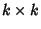## Characteristic Equation

The equation which is solved to find a Matrix's Eigenvalues, also called the Characteristic Polynomial. Given asystem of equations with Matrix(1)

the Matrix Equation is(2)

which can be rewritten(3)

M can have no Matrix Inverse, since otherwise(4)

which contradicts our ability to pick arbitraryand. Therefore, M has no inverse, so its Determinant is 0. This gives the characteristic equation(5)

wheredenotes the Determinant of A. For a generalMatrix(6)

the characteristic equation is(7)